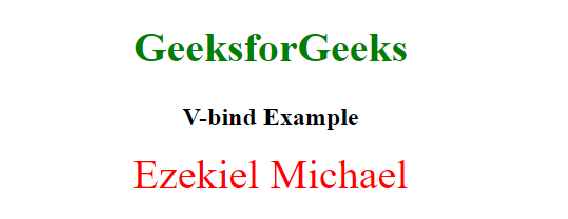Open in App
Not now

# Difference between v-bind and v-model in Vue.js

• Last Updated : 11 Apr, 2022

This article covers the difference between v-model and v-bind in Vue.js. The following steps are used to explain their differences.

The v-model is a two-way binding which means if you change the input value, the bound data will be changed. The v-model directive is used to create two-way data bindings on form input, textarea, and select elements.

Example:

## HTML

 ```<``html` `lang``=``"en"``>` `<``head``>``    ``<``meta` `charset``=``"UTF-8"``>``    ``<``meta` `http-equiv``=``"X-UA-Compatible"` `content``=``"IE=edge"``>``    ``<``meta` `name``=``"viewport"` `content``=``        ``"width=device-width, initial-scale=1.0"``>``    ``<``script` `src``=``"https://cdn.jsdelivr.net/npm/vue@2"``>``    ``<``title``>Difference between v-model and v-bind``    ``<``style``>``        ``.container {``            ``display: flex;``            ``justify-content: center;``            ``align-items: center;` `        ``}` `        ``p {``            ``padding-left: 20px;``        ``}` `        ``h1,h2 {``            ``text-align: center;``        ``}``        ``h1{``            ``color: green;``            ``font-size: 40px;``        ``}``    ````` `<``body``>``    ``<``section` `id``=``"app-vue"``>``        ``<``h1``>GeeksforGeeks``        ``<``h2``>V-Model Example``        ``<``div` `class``=``"container"``>``            ``<``input` `type``=``'text'` `v-model``=``'Message'` `/>``            ` `<``p``> Message: {{ Message}} `  `        ````    ``` `    ``<``script``>``        ``new Vue({``            ``el: '#app-vue',``            ``data() {``                ``return {``                    ``Message: ''``                ``}``            ``}``        ``});``    ````` ``

Output: This example means that if our data changes, our input too will change, and if our input changes, our data changes too.The v-bind is called one-way binding which means it binds our data one way. It can also be used to bind HTML attributes. This example shows a one-way data-binding using our style element using v-bind.

Example:

## HTML

 ```<``html` `lang``=``"en"``>` `<``head``>``    ``<``meta` `charset``=``"UTF-8"``>``    ``<``meta` `http-equiv``=``"X-UA-Compatible"` `content``=``"IE=edge"``>``    ``<``meta` `name``=``"viewport"` `content``=``        ``"width=device-width, initial-scale=1.0"``>``    ``<``script` `src``=``"https://cdn.jsdelivr.net/npm/vue@2"``>``    ``<``title``>Difference between v-model and v-bind``    ``<``style``>``        ``.container {``            ``display: flex;``            ``justify-content: center;``            ``align-items: center;``        ``}    ` `        ``h1,``        ``h2 {``            ``text-align: center;``        ``}` `        ``h1 {``            ``color: green;``            ``font-size: 40px;``        ``}``    ````` `<``body``>``    ``<``section` `id``=``"app-vue"``>``        ``<``h1``>GeeksforGeeks``        ``<``h2``>V-bind Example``        ``<``div` `v-bind:style``=``"headingText"``>``          ``Ezekiel Michael``       ````    ``` `    ``<``script``>``        ``new Vue({``            ``el: '#app-vue',``            ``data: {``                ``headingText: {``                    ``color: 'red',``                    ``fontSize: '40px',``                    ``textAlign:'center'``                ``}``            ``}``        ``});``    ````` ``

Output:Below are differences between v-model and v-bind

My Personal Notes arrow_drop_up